Precalculus : Solve and Graph Linear Inequalities

Example Questions

Example Question #1 : Solve And Graph Linear Inequalities

Solve and graph: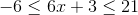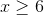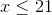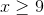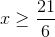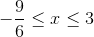Explanation:

1) Writeas two simple inequalities: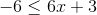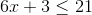2) Solve the inequalities: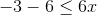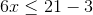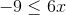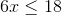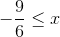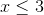3) Write the final solution as a single compound inequality:For interval notation: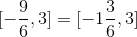4) Now graph: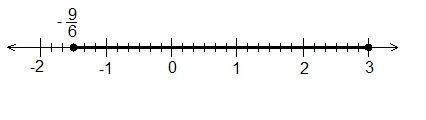All Precalculus Resources